# C++ Arithmetic Operators

Arithmetic operators carry basic arithmetic operations in a C++ program. These operators are used in simple mathematical expressions. They are also known as binary operators because each operator requires at least two operands.

Here is a list of arithmetic operators.

The operators plus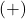, minus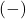, and multiplication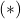need two numbers to produce results. Similarly, the divide operator requires two number –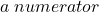and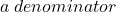. The result of the division is a quotient. The last arithmetic operator is modulo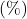which gives the remainder of a division of two integer numbers (not allowed for float or double types).

If one of the numbers is a floating point number and another integer for any arithmetic operator in C++ will result in a floating point value.

\begin{aligned}&integer + integer = integer\\ \\&float + integer = float\\ \\&integer + float = float\\ \\&float + float = float\end{aligned}

For example, the following program is a working example of the above rules.

### Program Code:

#include <cstdlib>
#include <iostream>
using namespace std;
int main()
{
// variable declaration

int a, b, result1;
float e,d, result2, result3, result4;

// Variable initialization

a = 20;
b = 30;

d = 25.5;
e = 20.3;

result1 = 0;
result2 = result3 = result4 = 0.0;

// Arithmetic Expressions

result1 = a + b;
cout << "a + b =" << result1 << endl;

result2 = a + d;
cout << "a + d =" << result2 << endl;

result3 = e + b;
cout << "e + b =" << result3 << endl;

result4 = d + e;
cout << "d + e =" << result4 << endl;

system("PAUSE");
return EXIT_SUCCESS;
}

## Output:

a + b = 50
a + d = 45.5
e + b = 50.3
d + e = 45.8

## Arithmetic Expressions

The operands and the C++ operators make an expression that evaluates to a single value. An expression can be a simple one with two numbers and a single operator or it can be a complex expression involving many numbers and operators.

From the above example,

\begin{aligned}
&e + b \hspace{3px}  evaluates \hspace{3px}  to \hspace{3px}  50.3\\\\
&d + e \hspace{3px} evaluates \hspace{3px} to \hspace{3px} 45.8
\end{aligned}

But, within the C++ program, an expression is written as

\begin{aligned}
&result3 = e + b\\ \\
&result4 = d + e
\end{aligned}

In a complex expression, the terms are evaluated based on the operator precedence. The expression with higher precedence operator is executed first and then the expression with lower precedence operator.

res = 2 + 4 * ( 1 + 5)

In the above example,

The parentheses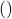has the highest precedence so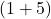is evaluated first.

The multiplicationhas second highest priority so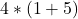is evaluated second.

The plus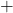is evaluated last.

\begin{aligned}
&= 2 + 4 * 6\\ \\
&= 2 + 24\\ \\
&= 26
\end{aligned}

## Example Program: Arithmetic Operators

#include <cstdlib>
#include <iostream>
using namespace std;
int main()
{
// Variable Declarations

int number1, number2, result1;
float number3, number4, result2;

// Variable Initialization

number1 = 100;
number2 = 74;
result1 = 0;

number3 = 24.65;
number4 = 12.5;
result2 = 0.0;

//Arithmetic Expressions

result1 = number1 + number2;
result2 = number3 + number4;
cout << "Integer Addition =" << result1 << endl;
cout << "Float Addition =" << result2 << endl;

result1 = number1 - number2;
result2 = number3 - number4;
cout << "Integer Subtraction =" << result1 << endl;
cout << "Float Subtraction = " << result2 << endl;

result1 = number1 * number2;
result2 = number3 * number4;
cout << "Integer Multiplication =" << result1 << endl;
cout << "Float Multiplication = " << result2 << endl;

result1 = number1 / number2;
result2 = number3 / number4;
cout << "Integer Division =" << result1 << endl;
cout << "Float Division = " << result2 << endl;

result1 = number1 % number2;
cout << "Integer Modulo Operation =" << result1 << endl;

system("PAUSE");
return EXIT_SUCCESS;
}

## Output:

The output of the above program is as follows.

Integer Addition =174
Integer Subtraction =26
Float Subtraction = 12.15
Integer Multiplication =7400
Float Multiplication = 308.125
Integer Division =1
Float Division = 1.972
Integer Modulo Operation =26